# Complex numbers Questions and Answers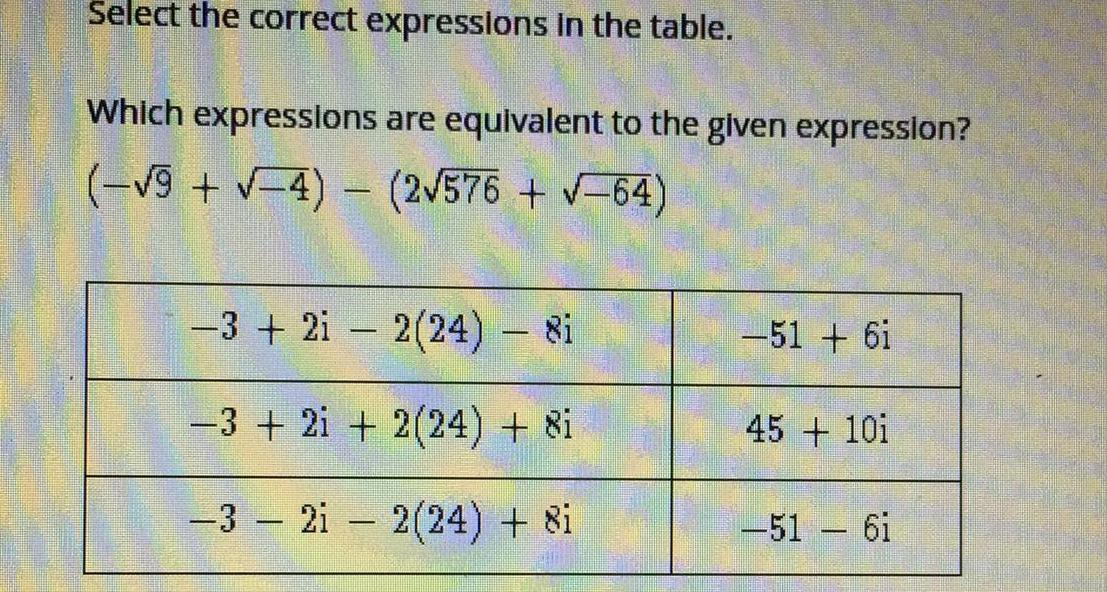Math
Complex numbers
Select the correct expressions in the table Which expressions are equivalent to the given expression 9 4 2 576 64 32i2 24 8i 3 2i 2 24 8i 32i2 24 8i 51 6i 45 101 51 6i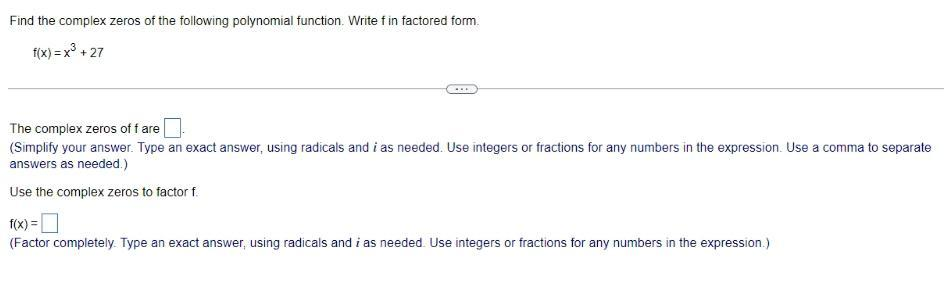Math
Complex numbers
Find the complex zeros of the following polynomial function. Write f in factored form. f(x)=x³ +27 The complex zeros of f are Use the complex zeros to factor f f(x) =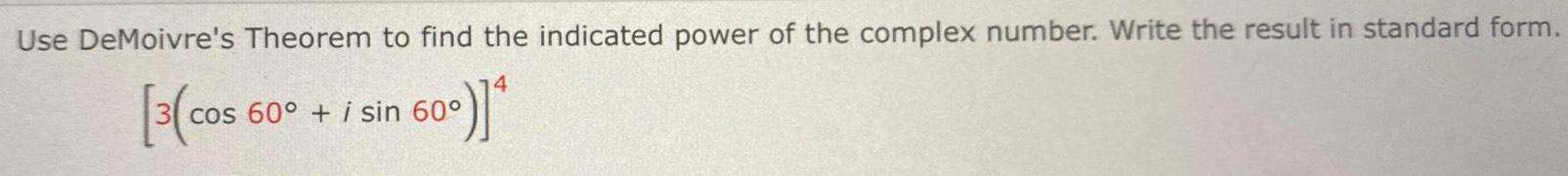Math
Complex numbers
Use DeMoivre's Theorem to find the indicated power of the complex number. Write the result in standard form.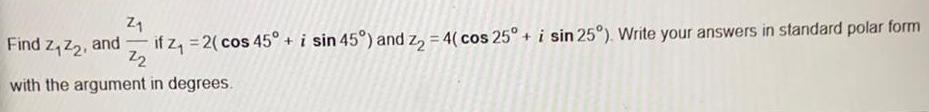Math
Complex numbers
Find Z₁ Z₂, and if z₁ = 2( cos 45° + i sin 45°) and Z₂ = 4( cos 25° + i sin 25°). Write your answers in standard polar form with the argument in degrees.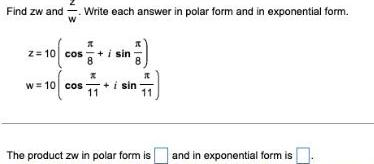Math
Complex numbers
Find zw and Write each answer in polar form and in exponential form. The product zw in polar form is and in exponential form is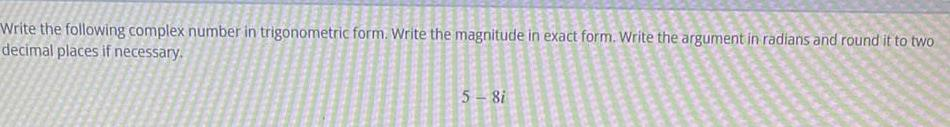Math
Complex numbers
Write the following complex number in trigonometric form. Write the magnitude in exact form. Write the argument in radians and round it to two decimal places if necessary. 5-8i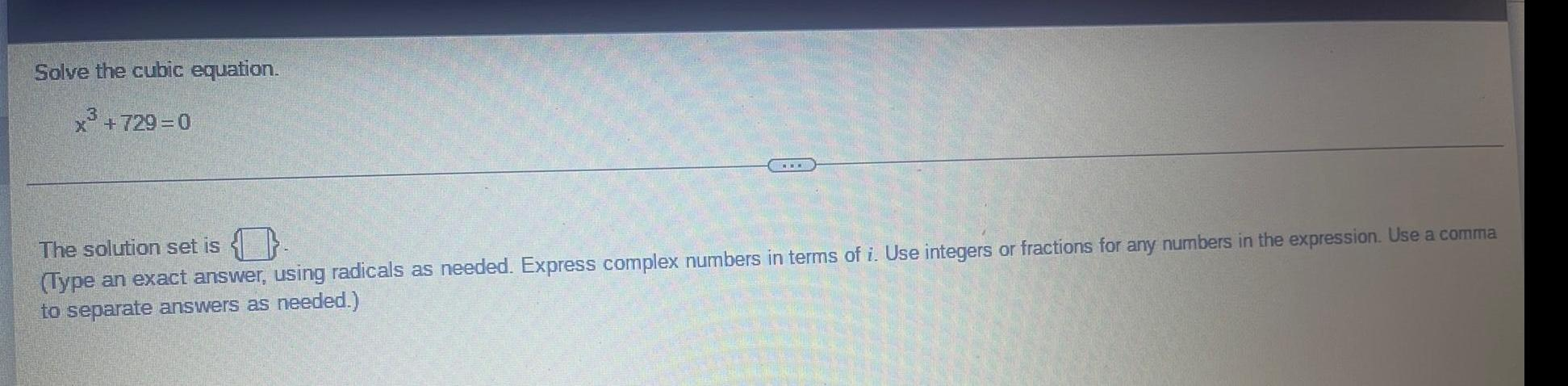Math
Complex numbers
Solve the cubic equation. x³ +729=0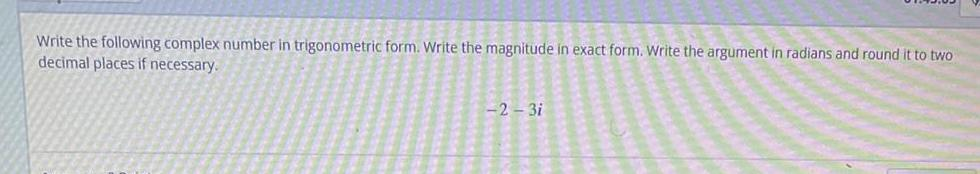Math
Complex numbers
Write the following complex number in trigonometric form. Write the magnitude in exact form. Write the argument in radians and round it to two decimal places if necessary. -2-3iMath
Complex numbers
Write the following complex number in standard form, z = a + bi. Write the exact answer. Do not round. 5 (cos (11π/6) + isin (11π/6))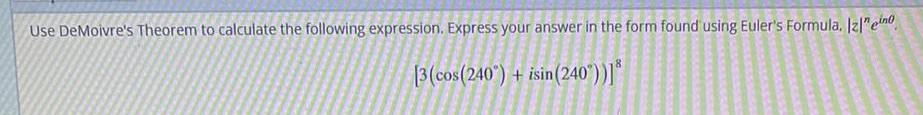Math
Complex numbers
Use DeMoivre's Theorem to calculate the following expression. Express your answer in the form found using Euler's Formula. |z|n ein0 [3 (cos(240°) + isin (240°))]8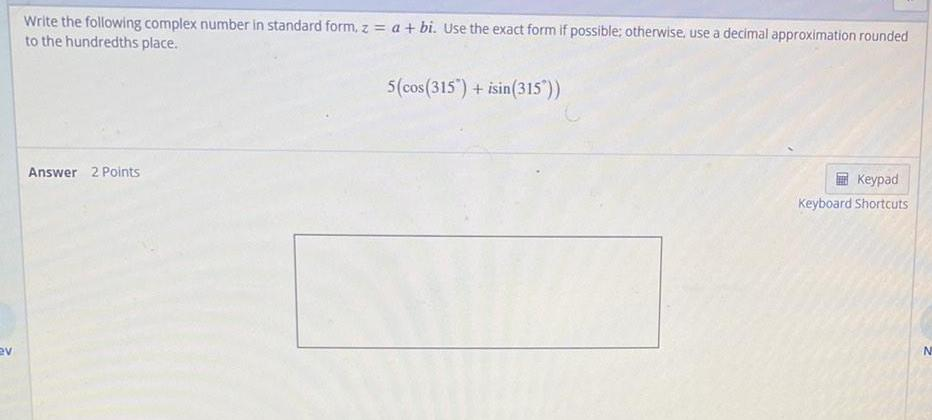Math
Complex numbers
Write the following complex number in standard form, z = a + bi. Use the exact form if possible; otherwise, use a decimal approximation rounded to the hundredths place. 5 (cos(315°) + isin (315°))Math
Complex numbers
Perform the following multiplication and write the product in trigonometric form. Express your result in the exact form, if possible; otherwise, use a decimal approximation rounded to the nearest hundredth. [4(cos(5π/4)+ isin (5π/4)][(4cos(3π/2)+isin(3π/2))] Solve the problem above and enter your solution below in trigonometric form. Recall that answers entered in degrees will require a degree symbol, which is located in the keypad.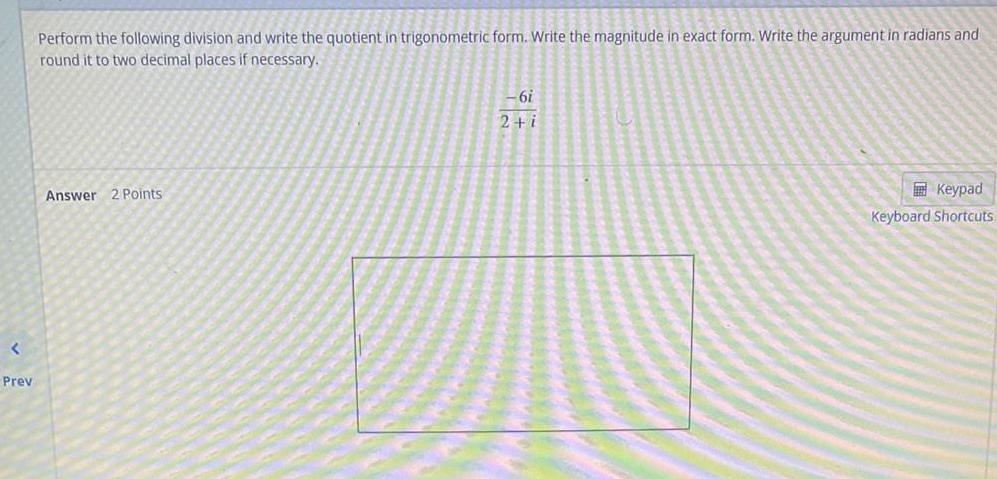Math
Complex numbers
Perform the following division and write the quotient in trigonometric form. Write the magnitude in exact form. Write the argument in radians and round it to two decimal places if necessary. -6i/ 2+i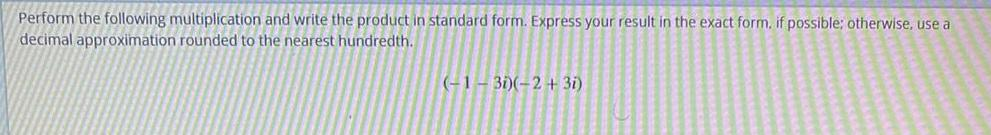Math
Complex numbers
Perform the following multiplication and write the product in standard form. Express your result in the exact form, if possible; otherwise, use a decimal approximation rounded to the nearest hundredth. (-1-3i)(-2+3i)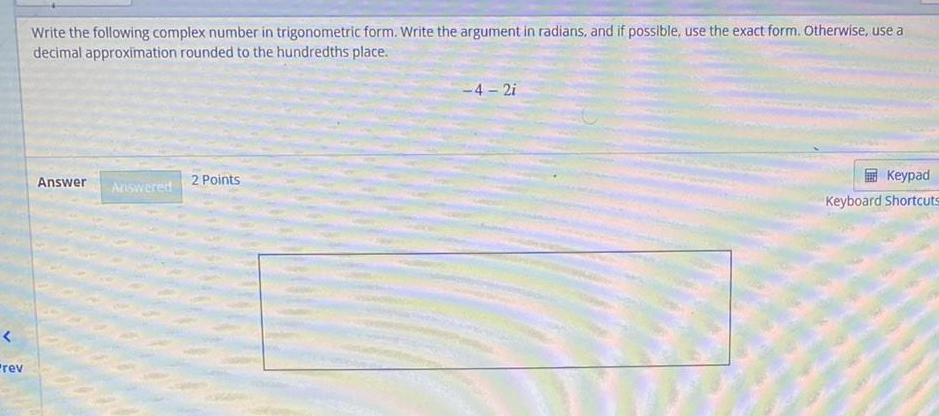Math
Complex numbers
Write the following complex number in trigonometric form. Write the argument in radians, and if possible, use the exact form. Otherwise, use a decimal approximation rounded to the hundredths place. -4-2i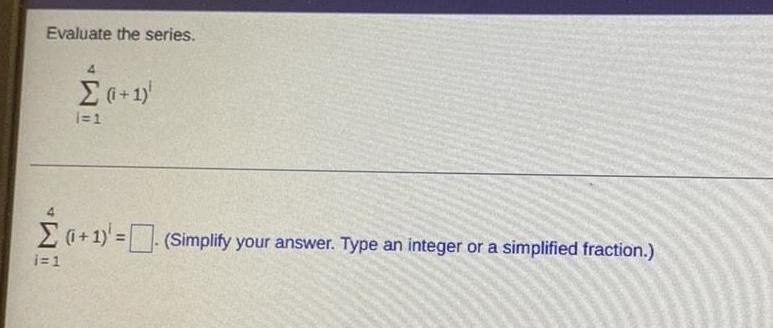Math
Complex numbers
Evaluate the series. 4Σ (i+1) i=1 i=14Σ (i+1) = (Simplify your answer. Type an integer or a simplified fraction.)